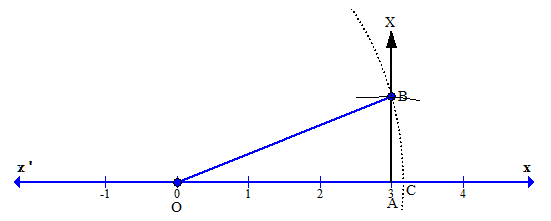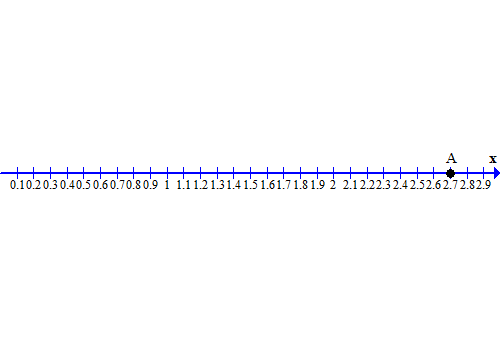Request a call back

<p>how to solve root 10 and 7.3 on number line</p> <p>&nbsp;</p>
Asked by gaurav72001 | 17 May, 2014, 03:22: PMExpert Answer
Consider a number line. Let the point O represent 0 and point A represent 3.
Now draw a perpendicular AP at A on the number line and cut off arc AB = 1 unit.
Using Pythagoras Theorem, we haveWith O as the centre andas radius draw an arc cutting real line at C. Clearly,.
Thus, C representson the number line.
Now let us find the square root of 7.3
Make a list of perfect squares, 0, 4, 9, 16, 25, 36, ....
Identify perfect squares closest to 7.3
Thus we have, 4 < 7.3 < 9
Take a positive square root of each number.
Thus, we haveNow evaluate the square roots.
Therefore, we have,Now make a list of perfect squares between 2 and 3.
 Numbers Squares 2.1 4.41 2.2 4.84 2.3 5.29 2.4 5.76 2.5 6.25 2.6 6.76 2.7 7.29 2.8 7.84 2.9 8.41
Thus, from the list it is clear that, 7.29 is closer to 7.3.
Therefore, we have,Thus, we can mark 2.7 on the number line as follows:Thus, OA represents the square root of 7.3
Answered by Vimala Ramamurthy | 21 May, 2014, 10:02: AM

## Concept Videos

CBSE 9 - Maths
Asked by jasaswinimallik | 04 Apr, 2022, 08:54: PMANSWERED BY EXPERT
CBSE 9 - Maths
Asked by shyammanoharjaiswal | 20 Jul, 2021, 08:12: PMANSWERED BY EXPERT
CBSE 9 - Maths
Asked by anilgupta9542 | 18 Aug, 2020, 02:41: PMANSWERED BY EXPERT
CBSE 9 - Maths
Asked by pandeyruby250 | 05 Jun, 2020, 07:24: PMANSWERED BY EXPERT
CBSE 9 - Maths
Asked by sarvankumar9124 | 06 May, 2020, 10:40: AMANSWERED BY EXPERT
CBSE 9 - Maths
Asked by Jaiwanthsiva | 04 May, 2020, 07:53: PMANSWERED BY EXPERT
CBSE 9 - Maths
Asked by Nimmi | 25 Apr, 2020, 01:38: PMANSWERED BY EXPERT
CBSE 9 - Maths
Asked by ajaypathak.ko | 10 Apr, 2020, 08:59: PMANSWERED BY EXPERT
CBSE 9 - Maths
Asked by srikantmpai | 05 Mar, 2020, 09:31: AMANSWERED BY EXPERT
CBSE 9 - Maths
Asked by dikshathakur1272005 | 29 Feb, 2020, 10:57: AMANSWERED BY EXPERT